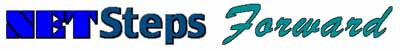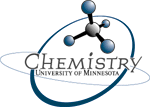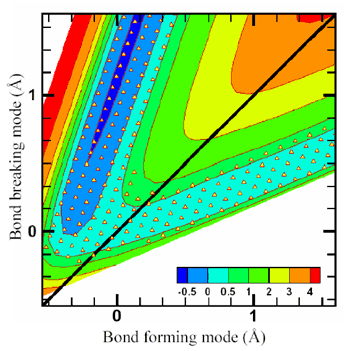## Comp Chem Research Developments

Archive of Comp Chem Research News
 March 2nd, 2005 Quantum mechanical reaction rate constants by vibrational configuration interaction Time-dependent quantum mechanics has a number of advantages for calculations of the reaction dynamics of polyatomic systems. It is especially efficient for generating results at a series of total energies, as required for thermally averaged rate constants, because a single wave packet carries information about a wide range of energies. Recent advances in the theory of vibrational configuration interaction method [A. Chakraborty, D. G. Truhlar, J. M. Bowman, and S. Carter, Journal of Chemical Physics 121, 2071 (2004), and references therein] have prompted a re-examination of the power of time-independent quantum mechanics, and now, for the first time, a converged quantum mechanical rate constant has been calculated for a polyatomic reaction by time-independent quantum mechanics. This work was carried out by graduate student Arindam Chakraborty and Professor Don Truhlar using the OH + H2 → H2O + H reaction as a prototype reaction to demonstrate the method. This application is a four-body system, but the method used here is not special to four-body reactions, and the results demonstrate that the time-independent vibrational configuration method can be a very convenient way to calculate converged quantum mechanical rate constants. Implementation of hierarchical expansion of the potential energy function in the present method will make the calculations affordable for larger systems. This work lays the groundwork for calculating converged rate constants for much larger reactions than have been treated up to now. The rate constant calculations were performed using flux autocorrelation functions, with a time-independent square-integrable basis set. Two modes that actively participate in bond forming and bond breaking were treated using two-dimensional distributed Gaussian functions, and the remaining (nonreactive) modes were treated using harmonic oscillator functions. The finite-basis eigenvalues and eigenvectors of the Hamiltonian were obtained by solving the resulting generalized eigenvalue equation, and the flux autocorrelation function for a dividing surface was represented in the basis formed by the eigenvectors of the Hamiltonian. The rate constant was obtained by integrating the flux autocorrelation function. An advantage of this procedure is that the vibrational configuration interaction method provides an intuitive picture of the mode coupling at the transion state while it also provides converged results. Furthermore, it suggests a number of well calibrated sublevels of theory that systematically approach the exact result; use of such sublevels for systematic convergence has been instrumental in the success of electronic structure theory but has so far played a lesser role in reaction dynamics.Fig. 1. Potential energy of the OH + H2 system as a function of bond-forming and bond-breaking modes, with all other degrees of freedom fixed at the saddle point geometry. (The other degrees of freedom are fixed for the plot, but not - of course - for calculating the rate constant). The contour spacing is 0.5 eV from 0 to 2 eV, then 1 eV from 2 to 5 eV. A dividing surface passing through the transition state of the reaction was defined such that it separates the reactant region from the product region; this dividing surface is shown as a solid line inclined at 45° to the axes. The reaction rate over a wide range of temperature (290–1100 K) was calculated by evaluating the net flux of particles across this surface from the reactants to the products arrangement. The location of centers of Gaussians basis functions are shown by solid triangles. Archive of Comp Chem Research News